A previous post, Performance is a Shape, Not a Number, describes how effective performance analysis tools let us explore the shape of our performance statistics through histograms and time series visualizations. Understanding performance in computer systems demands that operators be able to quickly investigate hypotheses about latency, throughput, and error ratios.

Sampling is widespread in observability systems because it lowers the cost of producing, collecting, and analyzing data in systems anywhere cost is a concern. Developers and operators in an observability system apply or attach key=value properties to observability data–spans and metrics–and we use these properties to investigate hypotheses about our systems after the fact. It is interesting to look at how sampling impacts our ability to analyze observability data, using key=value restrictions for some keys and grouping the output based on other keys.

Sampling schemes let observability systems collect examples of data that are not merely exemplary, but also representative. Sampling schemes compute a set of representative items and, in doing so, score each item with what is commonly called the item’s “sampling rate.” A sampling rate of 10 indicates that the item represents an estimated 10 individuals in the original data set.

Representivity is a statistical property that lets us construct approximate histograms and approximate time series to examine performance shapes using (sometimes) significantly less than all of the data, but there are two problems that commonly arise in working with sample data: (1) biased samples, and (2) too-small samples. As long as the sample is unbiased and not too small, we can use the collected sampling rates–i.e., representivity scores–on sample data to accurately summarize observability shapes for arbitrary key=value restrictions.

## Latency Quantile Time Series View

Let’s look at some typical data in an observability setting, first at full resolution, and then see how increased sampling lowers fidelity until the sample becomes statistically insignificant. Take a set of latency measurements from a stream of specific spans, for example, tagged by the machine that generated the measurement. In this example, we have a service with a fixed number of workers and variable service time, with some extra capacity to handle a burst of requests across six host machines. The overall latency of each request is impacted by queuing during a performance event, as seen in what is known as a latency quantile time series: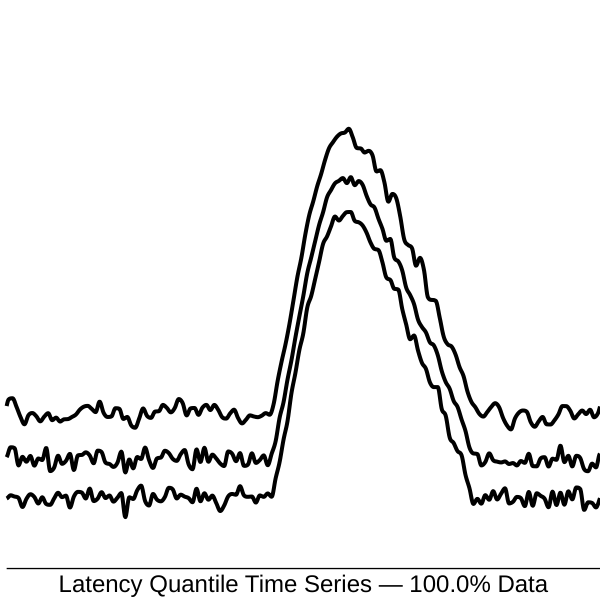In this diagram, time is on the horizontal axis and latency is on the vertical axis. Lines in the diagram above correspond with the 10%, 50%, and 90% latency quantiles. The 50% latency quantile, sometimes written “p50,” is also known as the median: half of the measurements are below and half are above the median value.

This time series shows what queueing looks like: latency climbs steeply when new requests arrive, but the service time, which is the amount of time spent by a worker (excluding wait time), looks unaffected. We can tell this is queuing, not a change in service time, because the spread between the p10 and p90 latencies is not affected during the event. Now let’s look at the same data using simple probability sampling, where items are selected for the sample with 10% probability.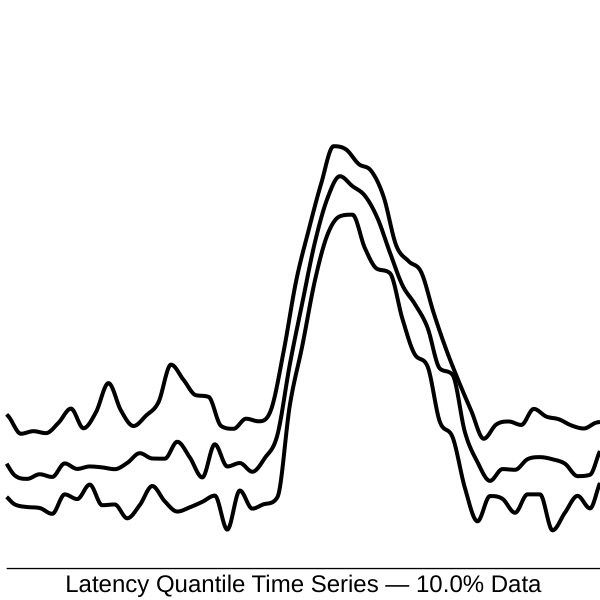It’s a close match. These curves reveal an accurate representation of the latency shape extrapolated from a 10% sample of the data, although the reduction does not come for free. Estimates drawn from the sample data have increased statistical variance. Sampled data gives “noisy” estimates, increasingly so the greater the sampling rate.

Here’s the progression of latency quantile time series at 100, 31.6, 10, 3.2, 1.0, 0.3, and 0.1% sampling probability, losing statistical significance to the right.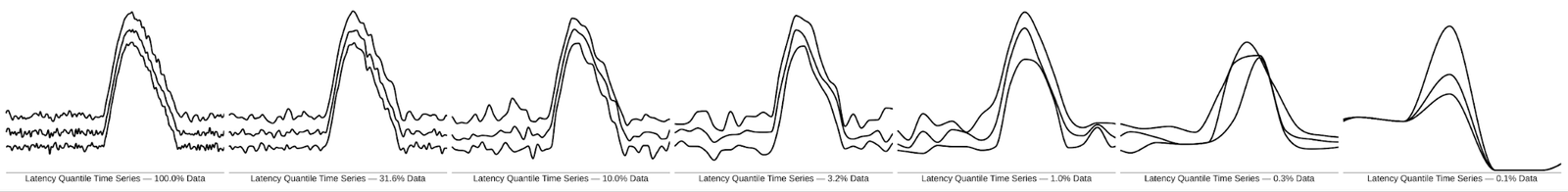## Latency Histogram View

To summarize an entire time window of latency values, we might instead view a latency histogram. In this diagram, latency is on the horizontal axis and the frequency of events is on the vertical axis. The latency distribution has two distinct modes, the first being service latency and the second being wait time. Continuing the example above, here is a view of the complete data: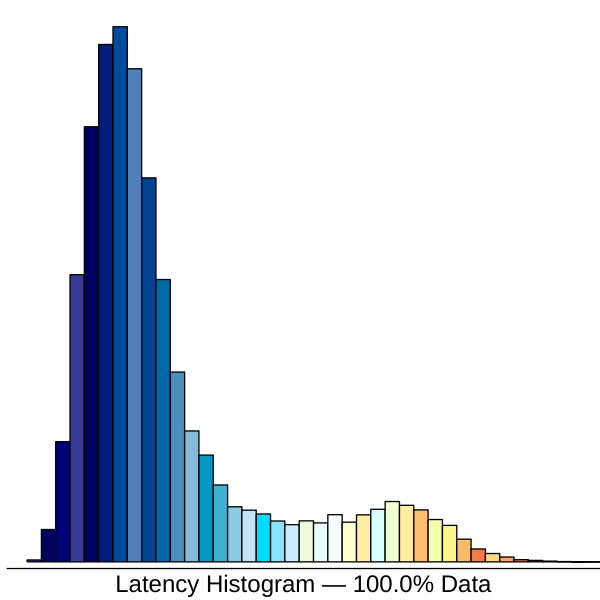Two modes can be seen in the latency histogram using data sampled at 10%, although not as distinctly: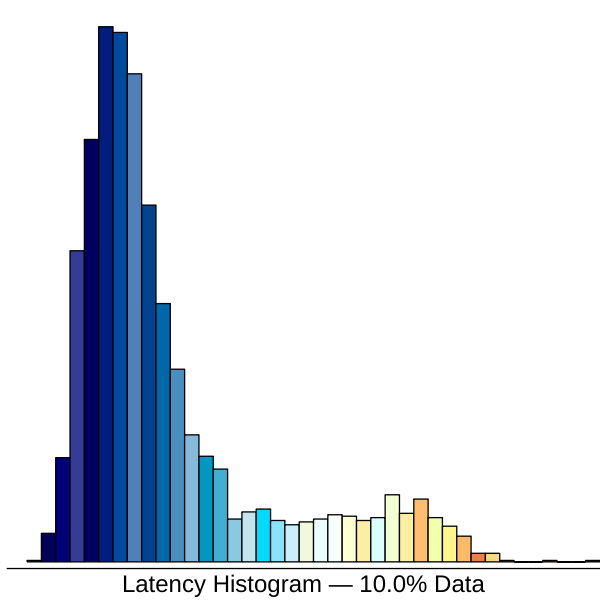Here is the progression of latency histograms, again from 100% to 0.1%: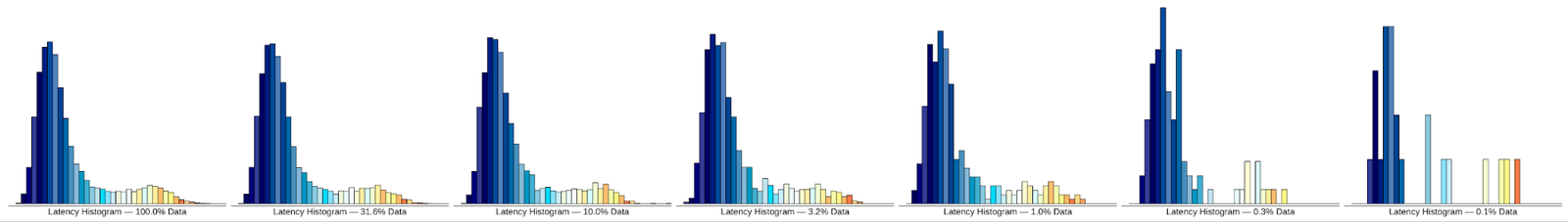It is important to realize, looking at an individual collection of sample data, that the data represents one out of many potential outcomes, had the random selection process gone differently. The unbiased property, mentioned above, implies that if we averaged out all the potential outcomes of a sampling scheme, they would average out to the true value. The sampling process used in this example has this property because each input has an equal and independent chance of being included in the output.

In this sampling scheme, technically called Bernoulli sampling, the probability of inclusion is the reciprocal of the sampling rate. It means if we accept items in the sample with 10% probability, the sampling rate for items in the output equals 10.

## Rate Time Series View

We can view the rate time series of requests using sample data as well. In this diagram, time is on the horizontal axis and frequency is on the vertical axis. The six hosts processing requests are given artificial colors. Here is the original rate data for the six machines viewed as a time series: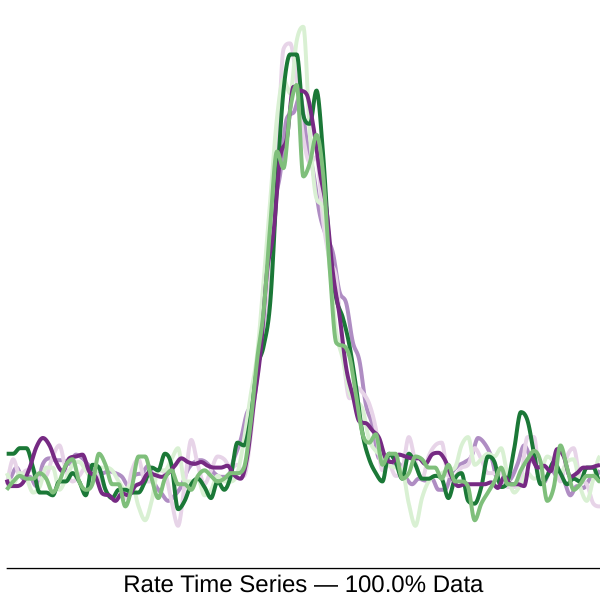We can see the cause of the overload event. The rate of requests rose equally across hosts due to a sudden burst of arrivals. Here is the same view for a 10% sample: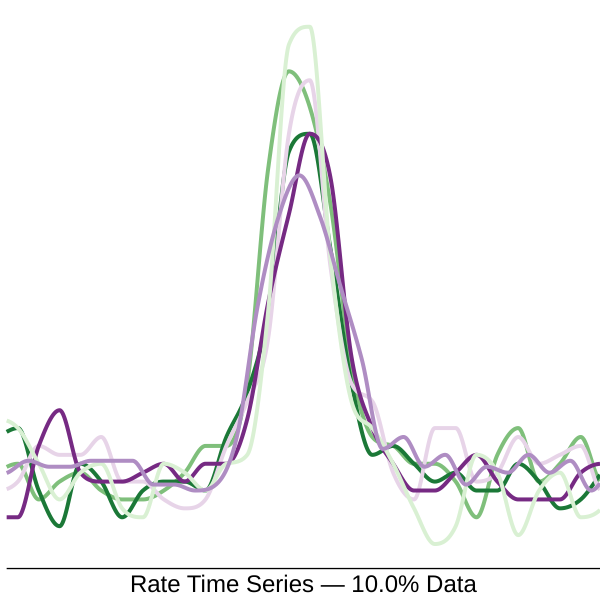Here is the progression of rate time series: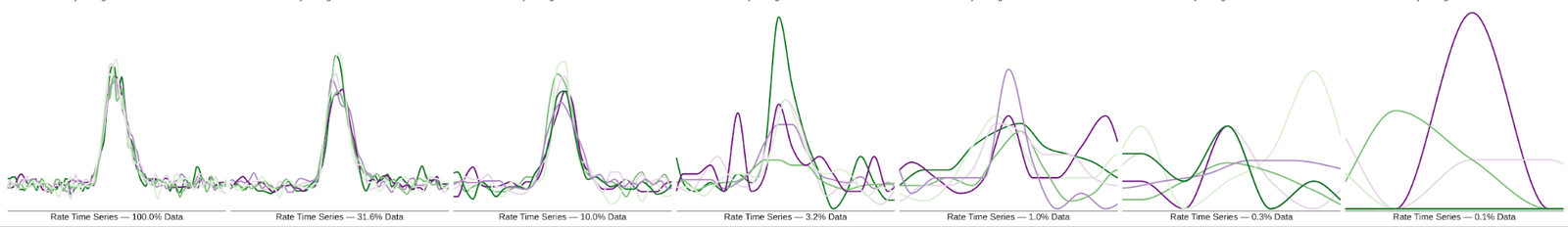When it comes to viewing time series of sampled data, we have a natural weapon against viewing samples that are too small. Because an unbiased sample averages out all possibilities, successive periods of data can be combined using a simple moving average.

The result is that even when the sample is too small to have statistical significance in a small window of time, we can simply enlarge the window of time and average the results to gain an accurate summary of the data.

## Rate Histogram View

We can confirm that servers received even load during the incident using a rate histogram by host value, indicated by color here. In this diagram, host value is on the horizontal axis and frequency is on the vertical axis. Here is the original data: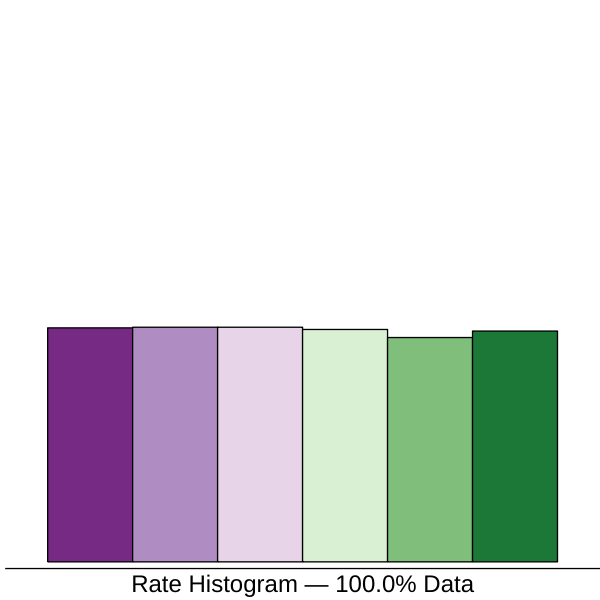This is a healthy looking shape. The six servers are processing an even amount of load, the outcome of uniformly-random load balancing across servers. Here is the same view for a 10% sample of the data: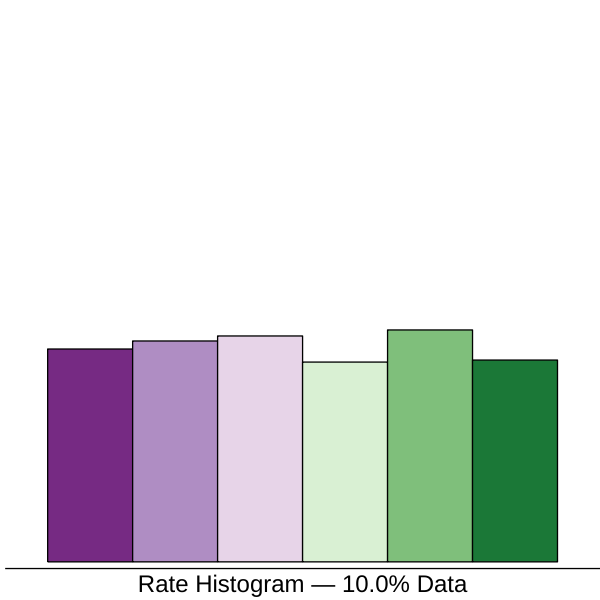This still looks approximately flat, great! Here’s the progression: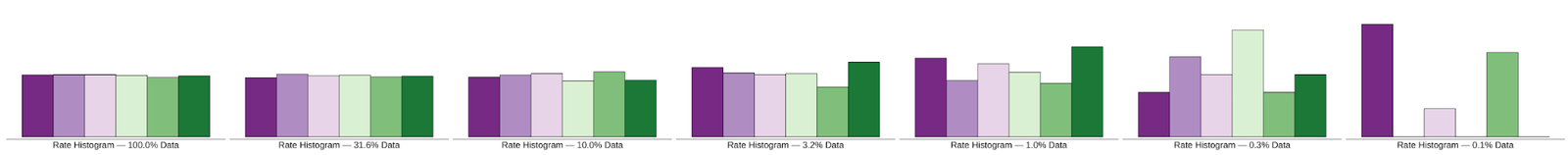Note that in the 0.1% sample on the right, there are only three values represented out of six. The 0.1% sample is more exemplary than it is representative of the complete data, as it lacks full “coverage” of the host dimension. The sample is definitely too small, in this case.

## Combined Latency and Rate View

Although it wasn’t stated above, the views seen so far were drawn using the same original data set and sample data sets. One sample generates all of the views we’ve seen. To illustrate how this will be applied in practice, consider the following process: (1) select a time window, (2) gather all samples collected spanning the window of time and drop items that fall outside the time window, (3) apply an arbitrary predicate and drop items that do not match, (4) render any of these views based on a subset of the sample data. Because the sample is unbiased, this works!

With a single data set generating multiple views, we might consider combining the information into a single view. Here’s one way to combine the two time series into one, for example. In this diagram, time is on the horizontal axis, latency is on the vertical axis, and per-host frequency is represented by an area of the corresponding color. Here is the original data, with latency quantiles showing: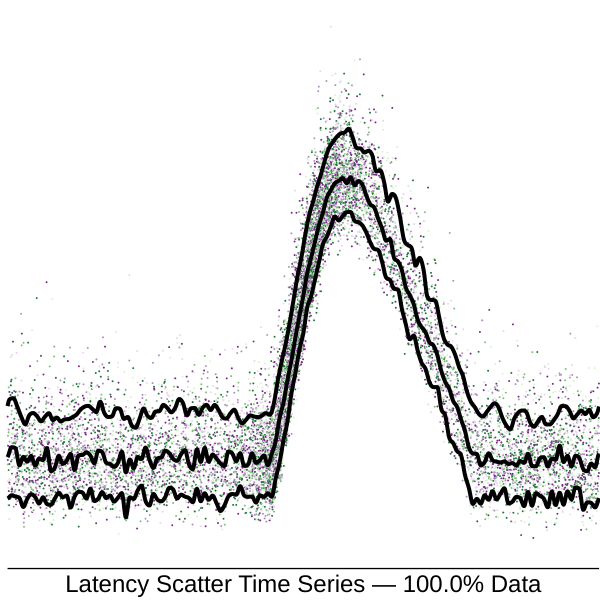The same view using a 10% sample: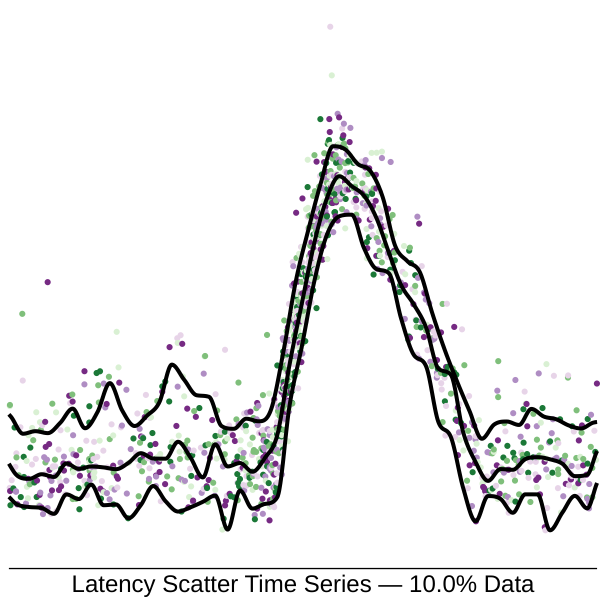Here is the progression of views from a 100% to a 0.1% sample:## And so much more…

By now you should have a grasp of the basic ideas behind sampling. Items of data are considered as they arrive and a subset (the “sample”) are kept with an associated representivity score, its “sampling rate.” A simple probability sampling scheme easily gives unbiased results, meaning we can view estimated histograms and time series for arbitrary key=value restrictions, as long as the sample is not too small.

Using sampling techniques, observability systems are able to display performance shapes at a fraction of the cost, compared with analyzing complete data. In the right configuration, sampling significantly reduces costs while having only a slight impact on data fidelity. With an unbiased sampling algorithm, we can summarize data after the fact using key=value restrictions. Unbiased sampling also ensures that if a sample is too small, we can “average out” the statistics over a larger window of time with accurate results.

In future posts on this topic, we’ll explore other ingredients in a sampling scheme, such as stratified sampling, weighted sampling, and reservoir sampling. How should we decide if a sample is too small? How can we prioritize good coverage? How should we apply these ideas in a tracing system with “head” and “tail” sampling facilities? How can we tackle high cardinality when sampling? Stay tuned!